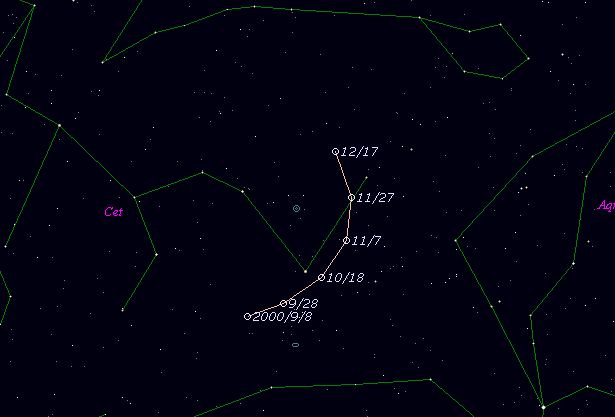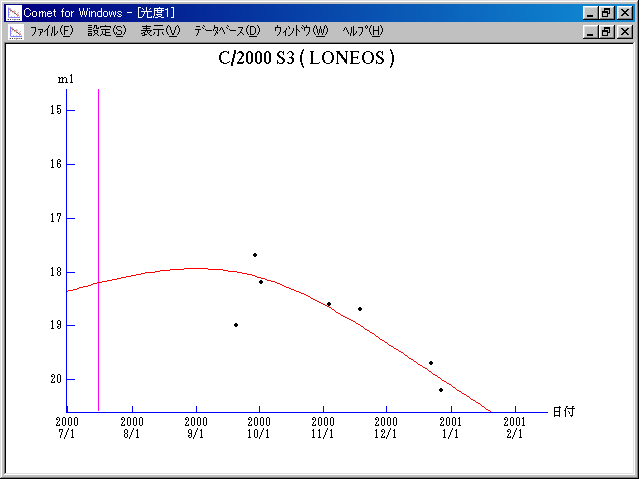# \$B%m%K%*%9WB@1(B

C/2000 S3 ( LONEOS )###\$B%W%m%U%#!<%k(B

 \$BH/8+F|(B 2000\$BG/(B9\$B7n(B29\$BF|(B \$BH/8+8wEY(B 17.7\$BEy(B \$BH/8+ B. A. Skiff (LONEOS)

###\$B50F;MWAG(B

```     T = 2000 July 15.867 TT          Peri. = 298.162
e = 0.77299                      Node  =  41.137   2000.0
q = 2.66273 AU                   Incl. =  25.179
a = 11.72976 AU     n = 0.024534     P =  40.17 years
```

###\$B@1?^(B###\$B8wEYJQ2=(B

```        m1 = 8.0 + 5 log\$B&\$(B + 20.0 log r
```##### \$B50F;MWAG\$O(B IAUC 7501 \$B\$K7G:\\$5\$l\$?\$b\$N\$G\$9!#(B \$B@1?^\$O(B StellaNavigator Ver.2.0 for Windows (\$B%"%9%H%m%"!<%D(B \$BJTCx(B / \$B%"%9%-!<=PHG6I4)(B) \$B\$G:n@.\$7\$?\$b\$N\$G\$9!#(B \$B8wEY%0%i%U\$O(BComet for Windows\$B\$G:n@.\$7\$?\$b\$N\$G\$9!#(B